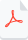# Abridged Riemann's Last Theorem Article

A Math Prize of \$10,000 or More

The Abridged Riemann's Last Theorem article is a condensed version of the original Riemann's Last Theorem article, which was originally longer and more detailed. In order to create a more concise version of the article, some parts were removed or modified. One such modification was the inclusion of the Identity theorem, which allowed the article to be shortened to just 6 pages. The Identity theorem is a fundamental concept in complex analysis that helps to establish the analytic continuation of a function, which is essential to the proof of Riemann's Last Theorem. By including this theorem in the abridged version of the article, readers can still gain a solid understanding of the proof while saving time and effort.Abridged Riemann’s Last Theorem.pdf